Understanding Non-uniform Forces in the Throws with Implications for Training

by

Wayne T. Armbrust, Ph.D.

Submitted in Partial Fulfillment of the Requirements for USA Track and Field Coaching Education Level III designation

I. Introduction

This paper, part of the requirement for Level III designation in the USA Track and Field Coaching Education program focuses on an area usually neglected in studies of the throwing events. Starting from basic kinematic and dynamic considerations involving non-uniform forces, we relate work done on an implement to the distance thrown, and graphically illustrate areas where specific training methods are likely to improve results.

II. Projectile Motion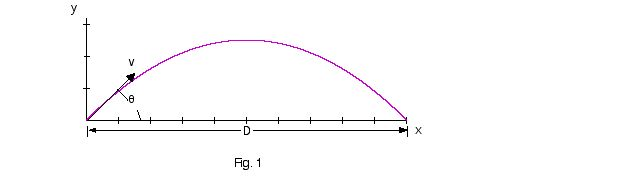We consider a projectile moving with an initial velocity v making an initial angle of ø with the ground. This will approximate an implement thrown with a velocity of release v and angle of release ø ( Fig. 1). We neglect considerations of air resistance, aerodynamic effects, and height of release, since to first order approximation these considerations will not effect our conclusions. Since distances traveled by thrown implements are small compared with the size of the earth, no error is introduced by assuming the acceleration due to gravity to be constant and neglecting effects of the curvature of the earth. From elementary physics8 it is easy to show that D, the distance traveled by a projectile under these assumptions is given by

D = (v2/g)sin(2ø). (Eq. 1)

where g is the acceleration due to gravity.

From Eq. 1 we see that sin(2ø) equals 1 when ø equals 45°, hence the maximum distance is achieved at this angle of release. This result is true only under the assumption of no air resistance or aerodynamic effects and not considering the height of release. It also assumes that the velocity of release is independent of the angle of release, which is not strictly true for a thrower. Air resistance, aerodynamic effects, and height of release all have the effect of making the optimal angle of release less than 45°, as does the fact that greater force can in general be generated at smaller angles of release. None of these considerations will effect the conclusions we are about to develop, however, since for a particular implement and athlete there will an optimal angle of release, øm, which will result in the maximum distance, Dm. Dm is given by

Dm = (v2/g)sin(2øm). (Eq. 2)

III. Work and Kinetic Energy

A. Uniform Forces For a body starting from rest and accelerating at a constant rate, the kinematic relationship between velocity, acceleration, and distance is give by

v2 = 2as, (Eq. 3)

where a is the acceleration and s is the distance over which the acceleration occurs.

For a constant force, Work , W, is defined as

W = Fs. (Eq. 4)

Substitution of Newton’s Second Law (F = ma) in Eq. 3 gives us

Fs = W = (1/2)mv2. (Eq. 5)

Here we follow the normal convention of representing vectors, quantities having direction as well as magnitude, in bold face.

The quantity (1/2)mv2 is defined as the kinetic energy. of an object. Eq. 5 expresses the very import relationship between work and kinetic energy; if an object starts from rest, it will have a final kinetic energy equal to the work done on it.

Work in the case of a constant force F acting through a distance s is graphically represented in Fig. 2 by the shaded rectangular area.

If we compare Eqs. 2 and 5 we obtain the result

Dm = (2W/mg)sin(2øm). (Eq. 6)

Thus, the distance an implement is thrown is directly proportional to the work done on the implement. No doubt many readers will have heard that distance thrown is proportional to the product of force and the distance through which the force acts. The derivation above is the origin of this statement, but is exact only for constant force.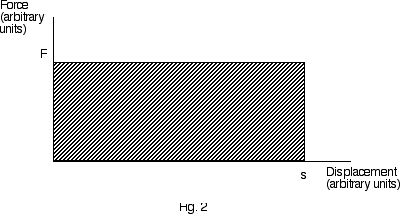B. Non-uniform Forces In nature, few forces are ever constant. This is especially true in track and field and the throws in particular. For a non-uniform force that varies in both magnitude and direction, the differential element of work is defined as

dW = F . ds = (Fcosø)ds (Eq. 7)

where F is the instantaneous force, ds is the differential element of displacement, and ø is the angle between F and ds. ds is always tangent to the displacement curve, which can be of arbitrary shape. Likewise, F can constantly change in direction and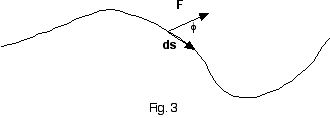magnitude. ø, the angle between the F and ds can also constantly change. A differential element of work is illustrated in Fig. 3. The differential work elements are integrated over the total displacement to calculate the total work.

A typical plot of Fcosø vs. s for a throwing event is shown in Fig. 4. Force, initially zero, builds to a maximum at an intermediate point and then decreases until release. The relationship between work and the area under the force vs. displacement curve from the previous section can be generalized to the case of non-uniform forces. For non-uniform forces, work is also the area under the Fcosø vs. s curve. Thus, we arrive a very important result: Increased throwing distances are equivalent to increased area under the Fcosø vs. s curve.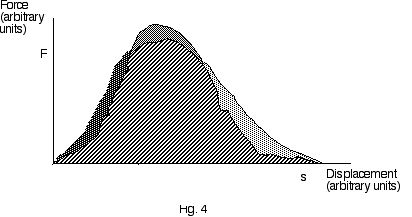IV. Implications

Having developed the relationship between work done on an implement and distance of the throw, we seek ways of increasing the area under the curve in Fig. 4. We will consider the area shaded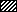to correspond to the work produced on the implement by the thrower, and hence proportional to the resulting distance, employing a base level of training. We now explore ways in which to increase the area under the curve in Fig. 4 beyond the base level.

Consider the portion on top of the curve shaded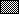.

We may think of this portion of the curve as resulting from improvement to peak force developed by the athlete. Peak force training is the most common type of strength training undertaken by throwers, and typically consists of training such as 1-3 sets of 3-5 repetitions of appropriate lifts at 75-95% SRM performed at relatively low speed12. Bompa1 recommends a maximum strength phase prior to training at a sport-specific combination of strength and speed. Unfortunately, many throwers, even those performing at a relatively high level, limit themselves by not going beyond this phase in their training. At this point we should note that the peak force developed in the throw will be less than the maximum force of which the athlete is capable. This is because the implement is moving at this point and it is well-known that the force of muscular contraction is inversely proportional to the speed of muscular contraction6. The force-velocity curve is illustrated in Fig. 5. Therefore, specific training for peak force in the throws should include training at moderate force - moderate speed of contraction4. Typical training of this type includes snatch and release or press and release exercises with moderate weight. Conventional training emphasizes only the left portion of the curve, i.e., large forces and low speeds.

Next let us consider the portion of the curve shaded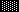at the initiation of the throw. This represents the increase in area under the force vs. displacement curve realized by increasing the initial slope of the curve, the rate of force development. Training to improve rate of force development should obviously be part of every thrower’s training program. If the total area under the force vs. displacement curve can be increased by even a few percent through improvement in this part of the curve, distance will correspondingly increase. Methods employed to improve rate of force development involve explosive, ballistic movements with moderate to heavy resistance, seeking to accelerate the weight as quickly as possible7,9,10, and plyometric training2.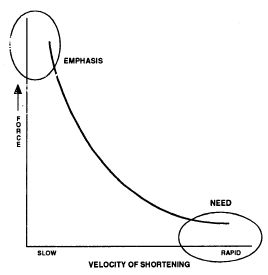Fig. 54

Finally we consider the right hand portion of the force vs. displacement curve in Fig. 4. This portion of the curve is characterized by a high rate of muscular contraction since it occurs just prior to release of the implement. Decrease of force with velocity, while retaining an inverse relationship, can be reduced through appropriate training. Training in the right hand portion of the curve of Fig. 5 will improve applied force at high speed4 and increase the area under the curve on the right hand portion of Fig 4. Specific training to increase force at high rates of muscular contraction include medicine ball and power ball drills5 and other high speed, low intensity resistance training13. Plyometrics are also shown to improve force production at high velocities3. The portion of the curve in Fig. 4 shaded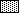illustrates improvement as a result of this type of training.

V. Conclusions

We have derived the relationship between the work done on an implement and the distance thrown, and have shown that to increase distance we must increase the area under the force vs. displacement curve. Those portions of the force vs. displacement curve where enhancement is most likely include increasing the rate of force development at the beginning of the throw and increasing force at high rates of muscular contraction before the release. Specific training suggestions for improvement in these areas include explosive, ballistic resistance training, high speed, low resistance exercises such as medicine ball training, and plyometrics.

VI. References

1. Bompa, T. O., Theory and Methodology of Training: The Key to Athletic
Performance
, 3rd Ed, Debuque: Kendall/Hunt Publishing Company, 1994.

2. Chu, D.A., Jumping into Plyometrics, Human Kinetics, Champaign, IL, 1991

3. Chu, D. (1983), Plyometrics: The Link Between Strength and Speed, National
Strength and Conditioning Association Journal, 5(2):20-21.

4. Gambetta, V. (1987), How Much Strength is Enough? National Strength and
Conditioning Journal,
9(3):51-53.

5. Gambetta, V., and Odgers, S., The Complete Guide to Medicine Ball
Training
, Sarasota, Florida: Optimum Sports Training, 1991.

6. Hill, A. V. (1953), The Mechanics of Active Muscle, Proc Roy Soc Lond (Biol),
141:104-117.

7. Newton, R. & Kraemer, W. (1994), Developing Explosive Muscular Power:
Implications for a Mixed Training Strategy, Strength and Conditioning,
16(5):20-31.

8. Resnick, R. & Halliday, D., Physics for Students of Science and
Engineering
, New York: John Wiley & Sons, Inc., 1960.

9. Sale, D.G. (1988), Neural adaptations to resistance training, Medicine and
Science in Sport and Exercise,
20:S135-S245.

10. Schmidbleicher, D. (1985), Strength Training Part I: Classification of Methods,
Sports Science Periodical on Research and Technology in Sport,
August 1985:1–12.

11. Siff, M. C. and Verkhoshansky, Y. V., Supertraining, Pittsburgh: Sports Science
Syndicate, 1996.

12. Stone, M., and O’Bryant, H., Weight Training: A Scientific Approach,
Minneapolis, Minnesota: Burgess Publishing Company, 1987.

13. Zatsiorsky, V. M., Science and Practice of Strength Training, Human
Kinetics, Champaign, IL, 1995.